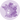# Hexbin map from geoJson file with Python

A hexbin map displays every region as a hexagone. This post shows how to load a `geoJson` file containing the hexagone coordinates and use it to build a map with python

## 📩 Load the `geoJson` file

This blogpost aims at building an hexbin map of US counties. Each county will be represented as a hexagone. The county boundaries are available here. For your convenience I've uploaded the exact file used in this post on github.

The coordinates are stored at `geoJson` format, probably the most common file format for this kind of information. If you want to know more about this, visit the background map section of the gallery that gives a tour on how to build a map with python.

Now, let's load this `geoJson` file from the web with `geopandas`

``````# Import geopandas library
import geopandas as gpd

url = "https://raw.githubusercontent.com/holtzy/The-Python-Graph-Gallery/master/static/data/us_states_hexgrid.geojson.json"
``````

That's it, we now have a geo dataframe containing all the counties as hexagones. Before starting to draw a map with it, you can have a look at it with:

``geoData.head(2)``
cartodb_id created_at updated_at label bees iso3166_2 google_name geometry
0 1219 2015-05-13T22:26:15+00:00 2015-10-05T16:00:16+00:00 Maine 60.5 ME Maine (United States) POLYGON ((-72.62574 55.31320, -69.90286 54.408...
1 1217 2015-05-13T22:24:35+00:00 2015-10-05T16:00:16+00:00 R.I. 47.8 RI Rhode Island (United States) POLYGON ((-72.62574 49.57439, -69.90286 48.544...

## 🙇 Basic hexbin map

`GeoPlot` is probably the best python library to build a map from a geo dataframe. It is pretty straightforward thanks to its `polyplot()` function.

``````# Import the geoplot library.
import matplotlib.pyplot as plt

# Set the plot size for this notebook:
plt.rcParams["figure.figsize"]=13,13

# Draw a map with matplotlib
geoData.plot(color="white", edgecolor='black', linewidth=.5);
plt.axis('off');``````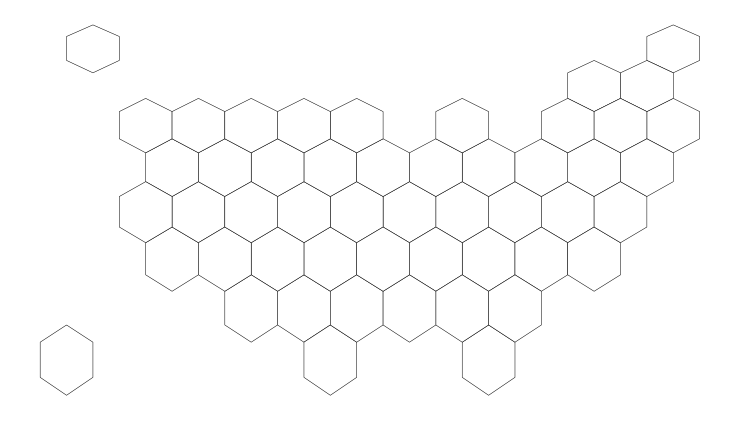## 🖊️ Add county names

Let's add county names at the centroid of each. Fortunately, centroids are already available from the `geometry` column of the geo dataframe. For instance, you can access it for the first county with `df.head(1).geometry.centroid`.

Let's add a `centroid` column to the original dataframe:

``````# add a "centroid" column with the centroid position of each county
geoData['centroid'] = geoData['geometry'].apply(lambda x: x.centroid)

# redraw the empty hexbin map:
geoData.plot(color="white", edgecolor='black', linewidth=.5);
plt.axis('off');

# for each county, annotate with the county name located at the centroid coordinates
for idx, row in geoData.iterrows():
plt.annotate(s=row['iso3166_2'], xy=row['centroid'].coords, horizontalalignment='center', va='center')
``````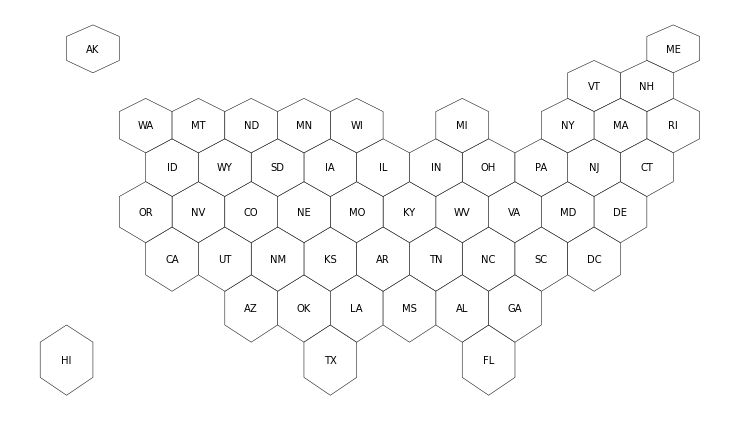Now let's plot both the county borders and their labels at centroids position.

## 🛠️ Load & merge numeric data

Let's transform the useless hexbin map above in a choropleth map, where each county is colored according to its mariage rate (# of mariage per 1000 inhabitants). The dataset is available here

``````# Read the mariage data stored on github:
import pandas as pd

# Add a new column to the geo dataframe that will be used for joining:
geoData['state'] = geoData['google_name'].str.replace(' \(United States\)','')

# Merge the mariage dataset with the geospatial information
geoData = geoData.set_index('state').join(mariageData.set_index('state'))
``````

It is now possible to create a first choropleth map as follow:

``geoData.plot(column="y_2015", cmap="viridis");``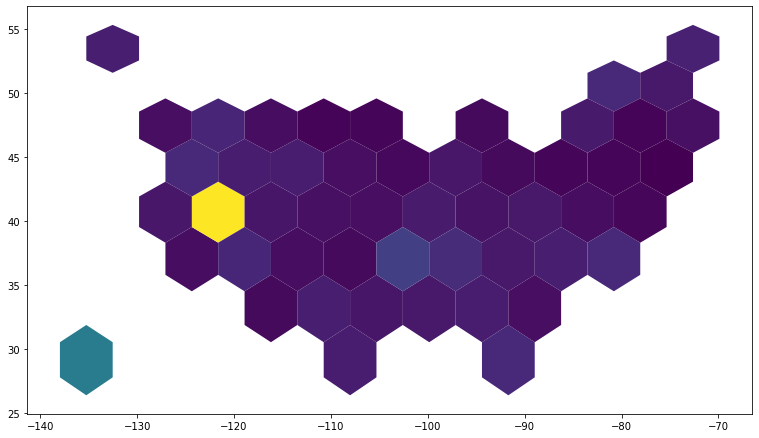## ✨ Final map

Let's add the final touch to make it look good. Title, annotation, better color scale and more:

``````# Initialize the figure
fig, ax = plt.subplots(1, figsize=(13, 13))

# map counties with the right color:
geoData.plot(
ax=ax,
column="y_2015",
cmap="BuPu",
norm=plt.Normalize(vmin=2, vmax=13),
edgecolor='black',
linewidth=.5
);

# Remove useless axis
ax.axis('off');

# Add title, subtitle and author
ax.annotate('Mariage rate in the US', xy=(10,440),  xycoords='axes pixels', horizontalalignment='left', verticalalignment='top', fontsize=14, color='black')
ax.annotate('Yes, people love to get married in Vegas', xy=(10,420),  xycoords='axes pixels', horizontalalignment='left', verticalalignment='top', fontsize=11, color='#808080')
ax.annotate('python-graph-gallery.com', xy=(600,0),  xycoords='axes pixels', horizontalalignment='left', verticalalignment='top', fontsize=8, color='#808080')

# for each county, annotate with the county name located at the centroid coordinates
for idx, row in geoData.iterrows():
ax.annotate(
s=row['iso3166_2'],
xy=row['centroid'].coords,
horizontalalignment='center',
va='center',
color="white"
)

# Add a color bar
sm = plt.cm.ScalarMappable(cmap='BuPu', norm=plt.Normalize(vmin=2, vmax=13))
fig.colorbar(sm, orientation="horizontal", aspect=50, fraction=0.005, pad=0 );
``````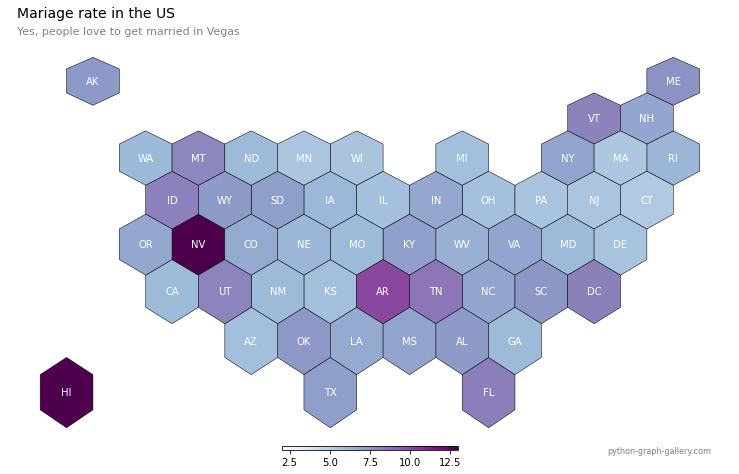Note: there are still some stuff that could be improved here but it's getting late 😴

## Contact & Edit

👋 This document is a work by Yan Holtz. Any feedback is highly encouraged. You can fill an issue on Github, drop me a message onTwitter, or send an email pasting `yan.holtz.data` with `gmail.com`.

This page is just a jupyter notebook, you can edit it here. Please help me making this website better 🙏!